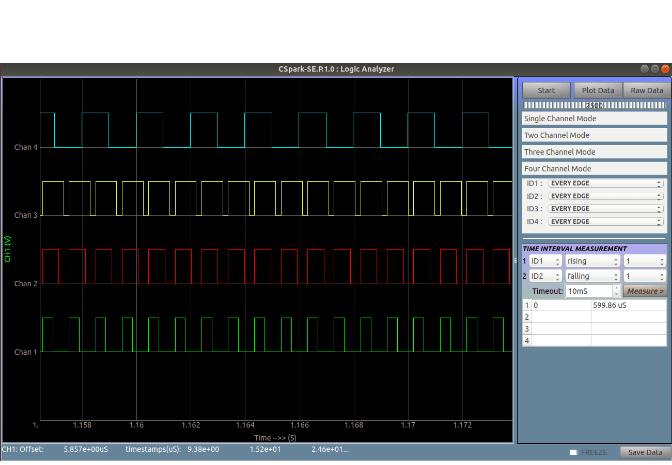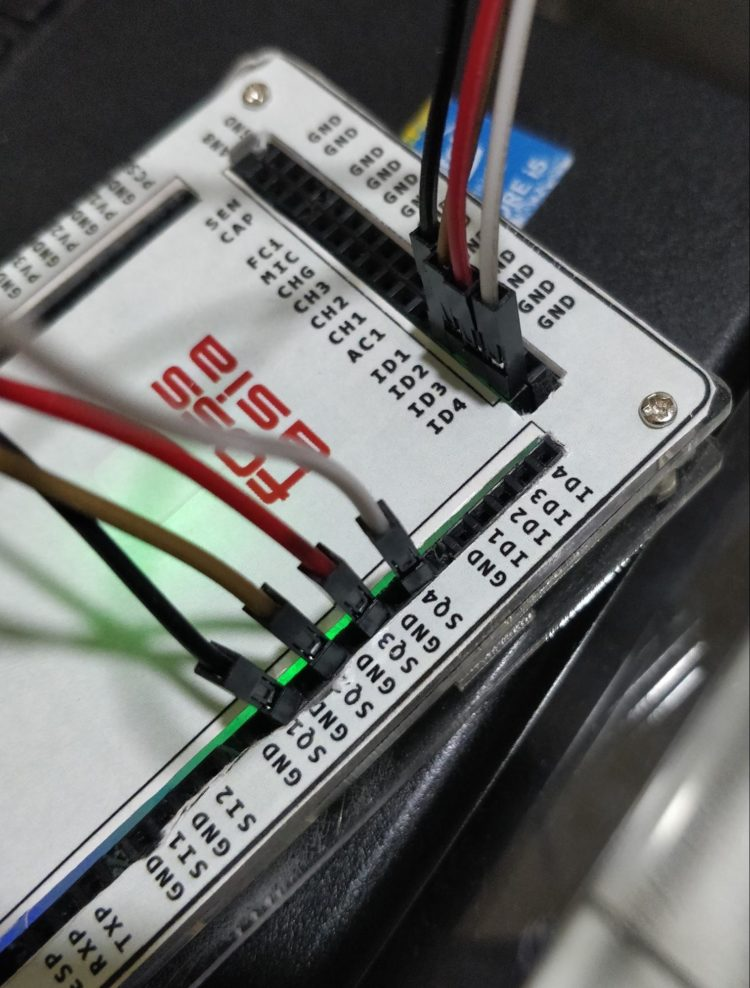# Working with Logic Analyzer in PSLab application

This blog demonstrates the working of Logic Analyzer instrument available in PSLab Desktop Application. It also includes a detailed description of the features available in the Logic Analyzer instrument. Also, it provides a step by step guide on how to work with the Logic Analyzer provided by PSLab which will be beneficial to first-time users.

## What is a Logic Analyzer?

A Logic Analyzer is an electronic instrument used to capture and display digital signals with an added functionality of providing the time difference between different edges of different pulses. It is mainly used to observe the time relationship between different digital signals. An example of a standard Logic Analyzer available in the market is as shown in figure 1.Figure 1. Standard Logic Analyzer

## How to generate different digital pulses in PSLab?

Logic Analyzer needs to be provided with some input of digital pulses among whom time relationship is to be found out. Digital pulses generated from different systems can be directly provided as input to the logic analyzer for analyzing. But PSLab provides a functionality to generate digital pulses up to some constrained frequency.

Following are the steps to generate different digital waves in the PSLab desktop application :

• Go to Advanced Control Section of PSLab app. The screen should look like one as shown in Figure 2.• PSLab device provides generation of maximum four digital waves at once. In this example, I will proceed by utilizing all the four pins i.e. SQR1, SQR2, SQR3, SQR4 (where SQR = Acronym of square wave generator and the number next to it is the pin ID available on the PSLab device). Set the duty cycles for each of the pins as desired (try to keep all the duty cycles different from each other to understand the process of measurement easily). After setting it should look something like Figure 3.Figure 3. Configuring PWM

NOTE: User can also set phase angle for different waves but I will proceed keeping all without any phase difference.

• Now set the frequency of the digital waves in the tab provided next to text Frequency and then press the SET button. This should generate desired digital waves when connected.

## How to analyze the generated waves in Logic Analyzer?

• Now go to the Experiments section and click on the Logic Analyzer instrument as shown in Figure 4.Figure 4. Test and Measurement Page

• Now a screen as shown in Figure 5 should open which is the main screen for Logic Analyzer Instrument.Figure 5. Logic Analyzer Main Page

On the right, you can see three buttons i.e Start, Plot Data and Raw Data. Below that selection for the number of channels is provided. And at last, the time measurement tool is provided which can measure the time difference between different edges of different digital waves. The graph at the center is the place where all the waves generated will be plotted.

• Now as we have generated four different waves, we need to navigate to Four Channel Mode in the Channel Selection section. There you can observe four pins i.e. ID1, ID2, ID3, and ID4 are selected by default as shown in Figure 6. So, we need to connect the pins SQR1, SQR2, SQR3 and SQR4 with pins ID1, ID2, ID3 and ID4 on the PSLab device.Figure 6. Channel Selection Section

NOTE: There are several options available for plotting the digital waves besides the one selected in the above image i.e.

1. Every Edge – Plot every edge of the signal
2. Falling Edges – Plot only falling edges of the signal (When a signal comes from 1 to 0 state)
3. Rising Edges Only – Plot only rising edges of the signal (When a signal comes from 0 to 1 state)
4. Disabled – Don’t plot the selected waveFigure 7. Connecting wires on PSLab device

• Connect the wires on the PSLab device as shown in Figure 7.
• Now, as the device is connected, we can use the three buttons located at the top right corner to plot the digital waves. The functionalities of all the three buttons are as follows:
1. Start – It collects exactly 2500 sample points from the wave generated to be plotted
2. Plot Data – It plots the data on the graph if the samples are collected successfully
3. Raw Data – It provides a sheet containing time at particular intervals and the difference between the data points at that particular time
• Press the Start button and as soon as the samples are collected, press the Plot Data button. Then the screen should look something like Figure 8.Figure 8. Final graph of input provided

Zoom in further, and the screen will look like Figure 9.Figure 9. Zoomed In graph for better visualization

• So now, the waves are plotted and are ready to be analyzed. Time difference between any two edges for any two distinct or a similar wave can be found out by using the TIME INTERVAL MEASUREMENT TOOL which is as shown in Figure 10.Figure 10. Time Interval Measurement Tool

• The working of this tool can be explained by taking an example as follow :Figure 11. Configuring the Time Interval Measurement Tool

Suppose time difference between the rising edge of ID1 and falling edge of ID3 is to be measured. So, set the parameters as shown in Figure 11 for the following situation.

The timeout of the Logic Analyzer can be set to any desired value from 10mS to 9999 mS. Here, I will proceed with 10mS of the timeout. Now, press the measure button to see the result. It will be same as the Figure 12 shown below if all the configurations are set as the one in the  example :Figure 12. Calculated time difference displayed

So in this way, many calculations can be taken out very easily and can be viewed at once at a single place without manually doing any calculations. Also, the data generated can be stored in the local system by pressing the Save Data button located at the bottom right corner.

## Resources

1. PSLab Desktop Application – https://github.com/fossasia/pslab-desktop-apps (Link to repo)
2. PSLab device pins sticker – https://github.com/fossasia/pslab-artwork/blob/master/Sticker/pslabdesign.png